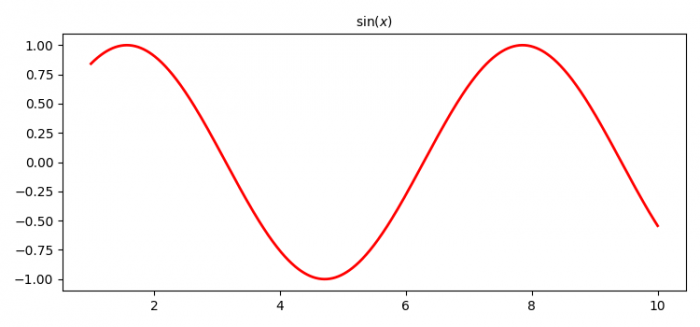# How to obtain the same font in Matplotlib output as in LaTex output?

To make bold font weight LaTeX axes label in matplotlib, we can take the following steps−

• Create data points for x.
• Create data points for y, i.e., y=sin(x).
• Plot the curve x and y with LaTex representation.
• To activate the label, use legend() method.
• To display the figure, use show() method.

## Example

import numpy as np
from matplotlib import pyplot as plt, font_manager as fm
fprop = fm.FontProperties(fname='/usr/share/fonts/truetype/malayalam/Karumbi.ttf')
plt.rcParams["figure.figsize"] = [7.50, 3.50]
plt.rcParams["figure.autolayout"] = True
x = np.linspace(1, 10, 1000)
y = np.sin(x)
plt.plot(x, y, label=r'$\sin (x)$', c="red", lw=2)
plt.title(label=r'$\sin (x)$', fontproperties=fprop)
plt.show()

## Output